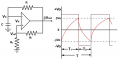# RC Time Constant

#### sparkie78

Joined Nov 5, 2016
21
I've been reading different articles about oscillators, or more specifically, multivibrators. To find the frequency, you divide 1 by the time constant of RC. I thought the formula for the time constant was simply R x C. But some articles said it was 2.2 x R x C or 2.3 x R x C or .693 x R x C or even 2 x pi x R x C. There might be others I haven't come across yet. Can someone explain this to me please? Thanks in advance

#### SamR

Joined Mar 19, 2019
4,548
The RC time constant t = RC. t is the product from calculating R x C. 5t is the time required to charge a capacitor (or nearly so as theoretically it never fully charges but gets closer and closer).

•TarikElec

#### WBahn

Joined Mar 31, 2012
28,177
Depends entirely on the circuit.

RC is the time constant associated with a resistor and a capacitor (typically in series). When a step change in voltage occurs, the capacitor voltage will go 1/e (where e is Euler's number -- about 2.718), which is about 63%, of the way from it's starting voltage to the voltage it will have eventually.

That time-dependent change in the capacitor voltage can be used in many different ways to create an oscillator.

In a 555 timer, the capacitor voltage changes between Vcc/3 and 2·Vcc/3 but the eventual voltages are 0 V and Vcc, respectively. If you do the math, the number 2.2 pops out.

#### crutschow

Joined Mar 14, 2008
31,524
But some articles said it was 2.2 x R x C or 2.3 x R x C or .693 x R x C or even 2 x pi x R x C. There might be others I haven't come across yet. Can someone explain this to me please?
Yes the value of the time-constant is fixed, but not the voltage on the RC charge/discharge curve where the multivibrator changes states.
Obviously, changing the tripping point voltage changes the multivibrator period.

For example, the ubiquitous 555, configured as a multi-vibrator, triggers at 1/3 of Vcc to turn on, and 2/3 of Vcc to turn off.
And the charge and discharge time-constants are often different.
So you can use that information, and the RC time-constant curve equation, to determine the on-time and off-time of the output signal.

So there is no one-size-fits-all equation for determining a multivibrators frequency from the RC values.

Last edited:

#### dl324

Joined Mar 30, 2015
15,511
Can someone explain this to me please?
We need to see schematics for the circuits.

#### MrChips

Joined Oct 2, 2009
28,133
Yes, time constant is R x C.

But that is not the same as time delay in a circuit or the reciprocal of frequency.
To find the time delay and hence frequency you need to know what portion of the charging and discharging waveform is being used to switch the ramp. As shown in the example below, T1 and T2 are not necessarily equal and not necessarily equal to R x C.#### AnalogKid

Joined Aug 1, 2013
10,222
To find the frequency, you divide 1 by the time constant of RC.
No. To find the frequency of a continuous waveform such as a sine wave or square wave, you divide 1 by the period of one complete cycle, whatever that may be.

I thought the formula for the time constant was simply R x C.
Correct.

But some articles said it was 2.2 x R x C or 2.3 x R x C or .693 x R x C or even 2 x pi x R x C.
Nope. Each of those is a real equation for a particular circuit. Nnone of them is an equation for the time constant, but each of them uses the time constant. Notice that each of the equations has the R-C value multiplied by a constant (2.2, 2.3, ln(2), 6.28). For example, the corner frequency of a single-pole highpass or lowpass filter is

1 / (2 x pi x R x C)

This also is the equation for the frequency of a Wien-bridge oscillator.

There are many different types of circuits that use one or more R-C networks to set the operating frequency in some manner, but that manner is dependent on the operating mode of the circuit.

ak

Last edited:

#### sparkie78

Joined Nov 5, 2016
21
No. To find the frequency of a continuous waveform such as a sine wave or square wave, you divide 1 by the period of one complete cycle, whatever that may be.

Correct.

Nope. Each of those is a real equation for a particular circuit. Nnone of them is an equation for the time constant, but each of them uses the time constant. Notice that each of the equations has the R-C value multiplied by a constant (2.2, 2.3, ln(2), 6.28). For example, the corner frequency of a single-pole highpass or lowpass filter is

1 / (2 x pi x R x C)

This also is the equation for the frequency of a Wien-bridge oscillator.

There are many different types of circuits that use one or more R-C networks to set the operating frequency in some manner, but that manner is dependent on the operating mode of the circuit.

ak
Got it. My mistake was thinking the time constant and the time period were the same thing. Thanks to everyone for their help. I really appreciate it.